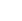# 李长河 (教授)Email：

# 实验十八 虚函数与多态、纯虚函数

一.实验目的

1.  在掌握继承与派生关系的基础上，进一步理解虚函数与多态性的关系，实现运行时的多态。

2.  学会定义和使用纯虚函数

1．范例：了解"单界面，多方法"的概念。现有称为figure的基类，存放了各二维对象（三角形、矩形和圆形三个类）的各维数据，set_dim()设置数据，是标准成员函数。

show_area()为虚函数，因为计算各对象的面积的方法是不同的。

【程序】

#include < iostream >

using namespace std;

class figure{

protected:

double x,y;

public:

void set_dim(double i,double j=0)

{  x=i;  y=j;  }

virtual void show_area()

{  cout<<"No area computation defined for this class.\n";

}

};

class triangle:public figure{

public:

void show_area()

{ cout<<"Triangle with height "<< x<<" and base "<< y<<" has an area of "<< x*0.5*y<< endl;

}

};

class square:public figure{

public:

void show_area()

{  cout<<"Square with dimensions "<< x<<" and "<< y<<" has an area of "<< x*y<< endl;

}

};

class circle:public figure{

public:

void show_area()

{  cout<<"Circle with radius "<< x<<" has an area of "<<3.14159*x*x<< endl;

}

};

int main(){

figure *p;

triangle t;

square s;

circle c;

p=&t;

p->set_dim(10.0,5.0);

p->show_area();

p=&s;

p->set_dim(10.0,5.0);

p->show_area();

p=&c;  p->set_dim(10.0);

p->show_area();

return 0;

}

【要求】

（1） 建立工程，录入上述程序，调试运行并记录运行结果。

（2） 修改上述程序，将virtual void show_area()中的virtual去掉，重新调试运行观察结果有何变化？为什么？

（3） 修改上述程序入口函数，使其动态建立三角形、矩形和圆形3个对象，通过基类指针访问这3个对象，然后释放这3个对象。

（4） 修改类定义中的析构函数，使之适应用户动态定义对    2、使用纯虚函数和抽象类对实验十六中的题1进行改进。

【要求】先定义一个抽象类Element,提供显示、求面积等公共接口（虚函数），派生出Point、Line、Circle等图形元素类，并重新定义（override）这些虚函数，完成各自的任务。

3、编写计算定积分的类。采用纯虚函数和抽象基类往往是建立一个类的框架，并非是要建立一个完整的类。要求首先定义定积分抽象基类basic_inte，